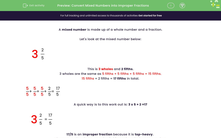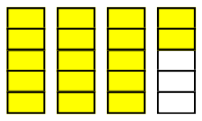# Convert Mixed Numbers into Improper Fractions

In this worksheet, students will practise converting mixed numbers into improper (or top-heavy) fractions.Key stage:  KS 2

Curriculum topic:   Number: Fractions, Decimals and Percentages

Curriculum subtopic:   Convert Fractions

Difficulty level:#### Worksheet Overview

A mixed number is made up of a whole number and a fraction.

Let's look at the mixed number below:

 3 2 5

This is 3 wholes and 2 fifths.

3 wholes are the same as 5 fifths + 5 fifths + 5 fifths = 15 fifths.

15 fifths + 2 fifths = 17 fifths in total.

 5 + 5 + 5 + 2 = 17 5 5 5 5 5A quick way is to this work out is: 3 x 5 + 2 =17

 3 2 = 17 5 5

17/5 is an improper fraction because it is top-heavy

That means that the top number is bigger than the bottom number.

e.g. convert the following mixed number into an improper fraction.

 5 4 7

Work out 5 x 7 + 4 = 39

 5 4 = 39 7 7